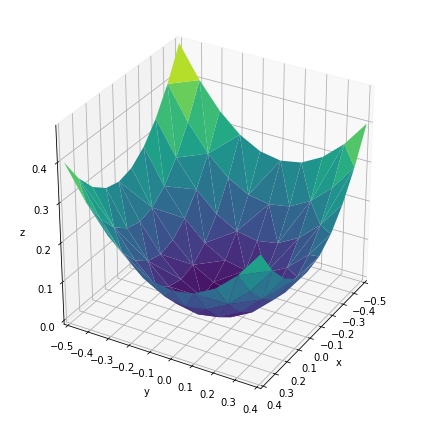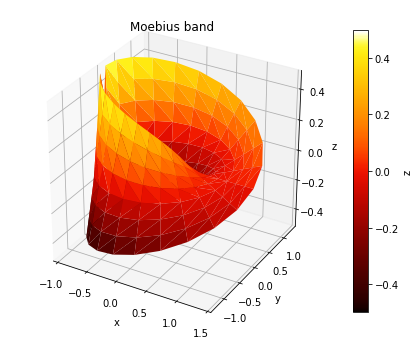# Trisurface ¶

Title
Trisurface Element
Dependencies
Matplotlib
Backends
Matplotlib
In :
import numpy as np
import holoviews as hv
hv.extension('matplotlib')The  Trisurface  Element renders any collection of 3D points as a surface by applying Delaunay triangulation . It is therefore useful for plotting an arbitrary collection of datapoints as a 3D surface. Like other 3D elements it supports  azimuth  ,  elevation  and  distance  plot options to control the camera position:

In :
%%opts Trisurface [azimuth=30 elevation=30 fig_size=200]
y,x = np.mgrid[-5:5, -5:5] * 0.1
heights = np.sin(x**2+y**2)
hv.Trisurface((x.flat,y.flat,heights.flat))

Out:Like all other colormapped plots we can easily add a  colorbar  and control the  cmap  of the plot:

In :
%%opts Trisurface [fig_size=200 colorbar=True] (cmap='fire')

u=np.linspace(0,2*np.pi, 24)
v=np.linspace(-1,1, 8)
u,v=np.meshgrid(u,v)
u=u.flatten()
v=v.flatten()

#evaluate the parameterization at the flattened u and v
tp=1+0.5*v*np.cos(u/2.)
x=tp*np.cos(u)
y=tp*np.sin(u)
z=0.5*v*np.sin(u/2.)

surface = hv.Trisurface((x, y, z), label='Moebius band')
surface

Out: